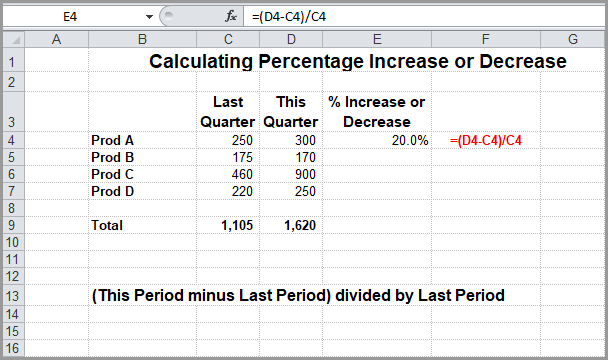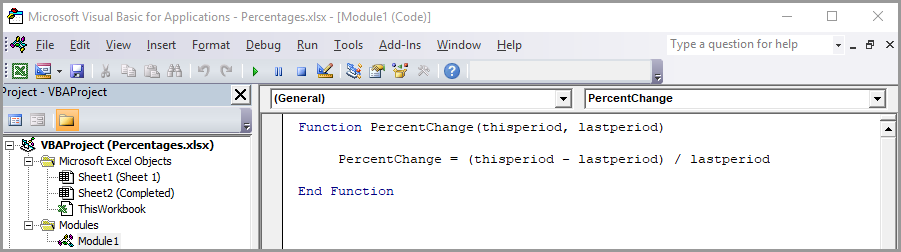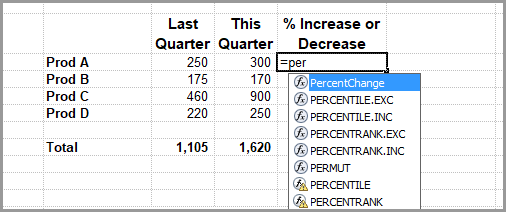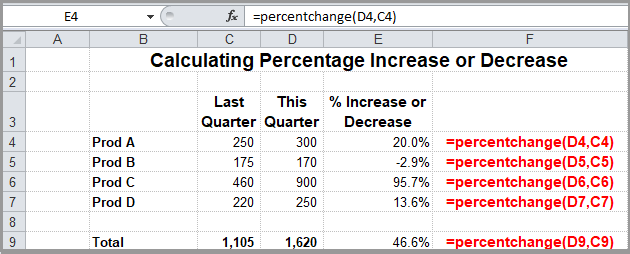Select Page

Share

As of Excel 2016 there are 467 functions, but the designers couldn’t possibly anticipate every user’s calculation needs. So Excel provides you with the ability to create your own custom functions.

Custom functions use the Visual Basic for Applications (VBA) programming language. But you don’t have to be a programmer to create your own function. Excel functions perform calculations instead of taking actions. So if you know how to create a formula you can probably convert it into a function.

Let’s say that you customarily have to calculate the percentage increase or decrease from one period to the next. For example, you have sales figures from last quarter and sales figures from this quarter and you want to see if they’ve gone up or down.  In the following spreadsheet this is done with a formula.I can’t tell you over the years how often I had to look up this statement:

(This Period minus Last Period) divided by Last Period

Was it (this period minus last period) divided by this period?  Was it (last period minus this period) divided by this period?  You get the picture.

A function did the trick.

If you want to create a function, press Alt+F11 to open the Visual Basic Editor, which opens up in a new windows. Then click Insert > Module. A new module window appears on the right-hand side of the Visual Basic Editor.

The code for my function looks like this:

Function PercentChange(thisperiod, lastperiod)

PercentChange = (thisperiod – lastperiod) / lastperiod

End Function

Each function begins with the word “Function” followed by the name you’ve given to your custom function.  In the case, I called it “PercentChange.”  After the function name you place in parentheses the values you need to calculate the result.  In this case there are only two:  the value for this period and the value for last period, in that order.  You just have to remember to refer to the most recent period first.

The line or lines following just describe how the function should be calculated.

The last last should always read “End Function.”

Here’s how it looks in the Visual Basic Editor.Now in the spreadsheet instead of entering the formula that was in the first image, you use the new PercentChange function.  When you start entering your function, Excel is nice enough to include it with all of the built-in functions and tries to help you with it.You drag to copy it down just like any other formula.  Here it is filled in.